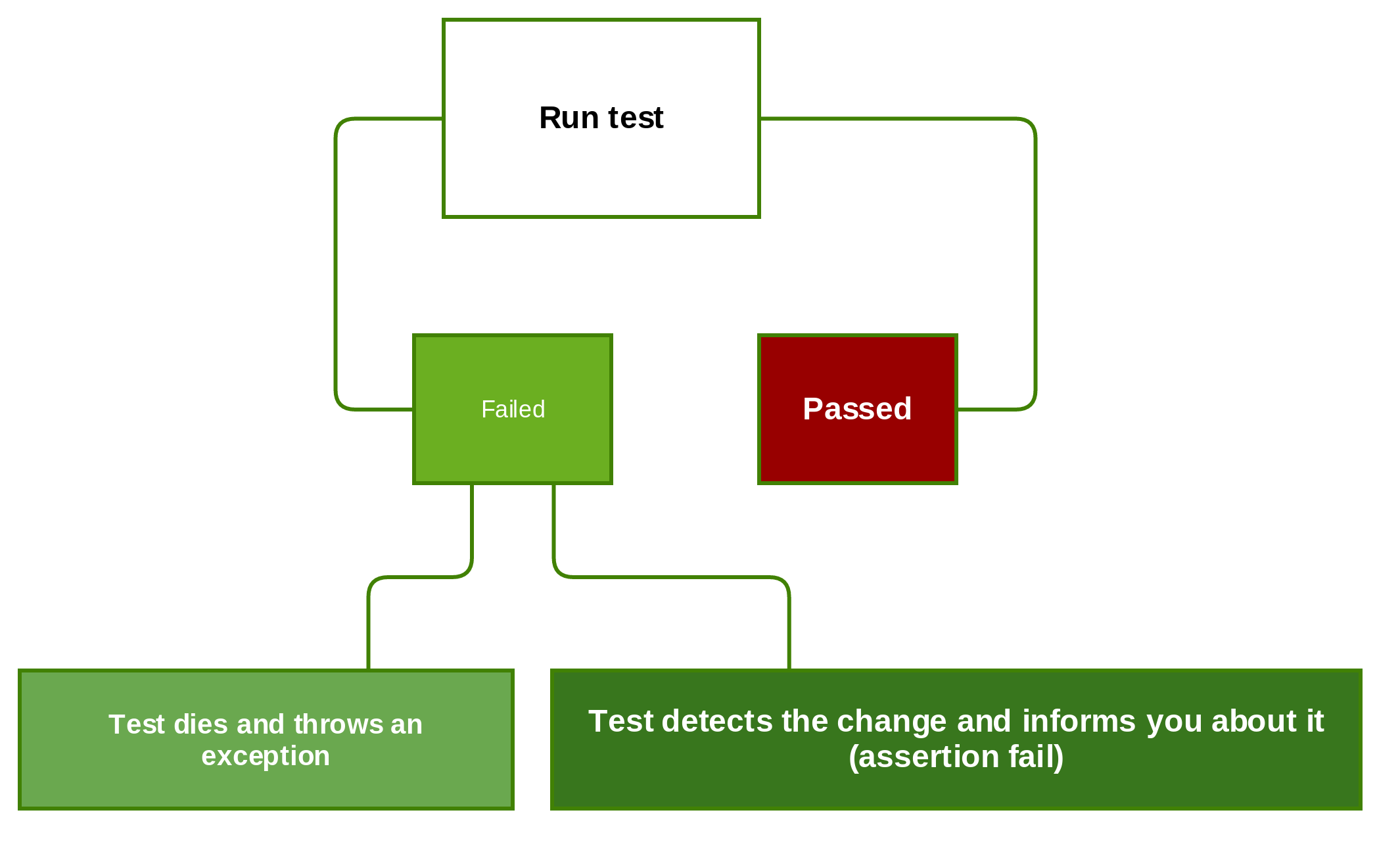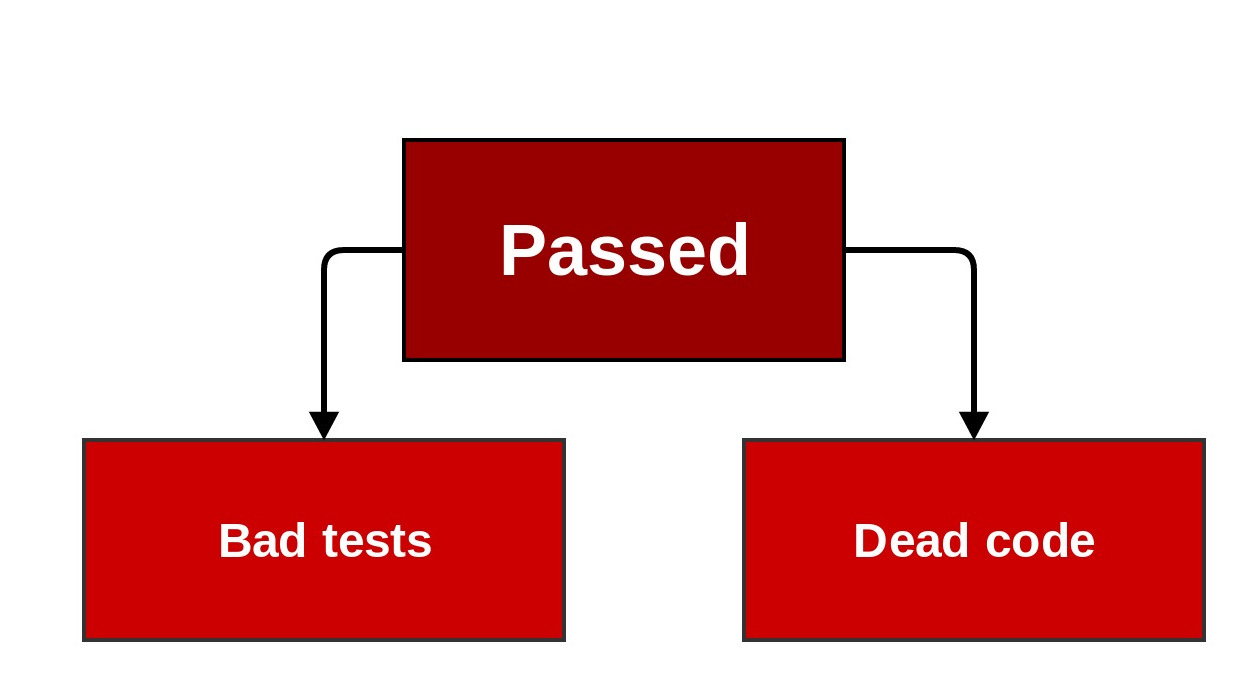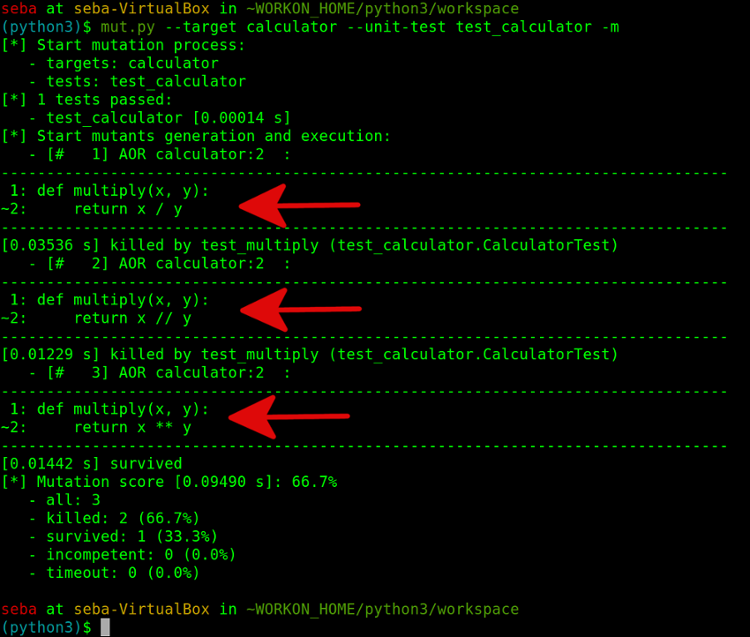## Mutation Testing

##### or who is going to test your tests ?

1st Developers@CERN Forum

Created by Sebastian Witowski

## Do you test your tests ?## Do you test the tests for your tests ?## Testing tests ?Richard Lipton, Fault Diagnosis of Computer Programs, 1971

## Step 1. Change you code in a small way:

• a + b ---> a * b
• a + 1 ---> a + 2
• a + b ---> a + c
• a + 1 ---> a + 1
a + 1
• (if a == 1 and b > 1) ---> (if a == 1 or b > 1)

Changes similar to small, programming errors.

## Step 2. Run your tests## Step 2. Run your tests## Step 3. Get the mutation score

Mutation score =
number or mutants killed
number of mutants created

# Example time

``````def multiply(a, b):
return a * b``````

``````def multiply(a, b):
return a * b``````

``````class CalculatorTest(TestCase):
def test_multiply(self):
self.assertEqual(multiply(2, 2), 4)``````

``self.assertEqual(multiply(2, 2), 4)``
``self.assertEqual(multiply(3, 3), 9)``## Equivalent Mutant Problem

``````index = 0
while True:
do_stuff()
index = index + 1
if index == 10:
break``````

if index == 10:
vs.
if index >= 10:

## Wrap up## The good parts

• Detect problems with your tests
• They are automatic
• How else would you test your tests ?
• (Semi-)Automatic tool for testing ? I'm in !

## The not so good parts

• Mutation testing is slow:
(TIME = ALL MUTANTS x ALL TESTS)
• Handful of libraries
• Equivalent Mutant Problem
• Writing complex mutant tests is difficult

## Mutant testing libraries

• Mutant - Ruby (last updated September 2015)
• VisualMutator - C# (last updated September 2015)
• Pitest - Java (last updated August 2015)
• Humbug - PHP (last updated May 2015)
• MuCheck - Haskell (last updated January 2015)
• MutPy - Python3 (last updated January 2014)
• Mutator - commercial solution for Java, Ruby, JavaScript and PHP

## Example

MutPy (requires Python3)

calculator.py

``````def multiply(a, b):
return a * b``````

test_calculator.py

``````from unittest import TestCase
from calculator import multiply

class CalculatorTest(TestCase):
def test_multiply(self):
self.assertEqual(multiply(2, 2), 4)````````self.assertEqual(multiply(2, 2), 4)``
``self.assertEqual(multiply(3, 3), 9)``## Any questions ?

Happy coding testing !

This presentation is available on github, so you can see the slides on github pages